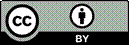Article citation information:

Nemchinov, S., Khristenko, A. Stress-strain state of pneumatic flexible shaft coupling for ball mill drives. Scientific Journal of Silesian University of Technology. Series Transport. 2018, 99, 125-134. ISSN: 0209-3324. DOI: https://doi.org/10.20858/sjsutst.2018.99.12.

Serge NEMCHINOV, Alexander KHRISTENKO

STRESS-STRAIN STATE OF PNEUMATIC FLEXIBLE SHAFT COUPLING FOR BALL MILL DRIVES

Summary. The article explores the stress-strain state of the pneumatic flexible shaft coupling of the tumbling mill drive using a software of finite element analysis. The study has revealed that the stress-strain state of the pneumatic flexible shaft coupling is characterized by an uneven general and local distribution of stresses. Areas of maximum stress and strain in the pneumatic flexible shaft coupling have been defined. The study allowed for changing the geometry and reducing the mass of the disc of the pneumatic flexible shaft coupling with a slight change in stresses and strains. The results of the study can be applied to the design of pneumatic flexible shaft couplings and serve as a basis for further research.

Keywords: pneumatic flexible shaft coupling; finite element method; geometry; stress; strain; displacement

1. INTRODUCTION

Various couplings that serve to transmit rotary motion from one shaft to another are widely used in many industries. Drive couplings may also perform other important functions: compensation for small mounting misalignments of units and assemblies, disconnection of shafts, automatic control of machine operation, smooth coupling of shafts on machine start-up, protection of machines from breakdowns in emergency mode etc . In today’s mechanical engineering context, couplings with non-metal flexible elements, which possess high compensating and damping properties, are widely used . However, these couplings are generally applied for the transmission of low and medium torque because of the low strength of rubber and polymers. The studies by Vinogradov and Homisin show that pneumatic flexible shaft couplings are free from these disadvantages and can transmit high torques with the possibility of adjusting rigidity within a wide range [3-7]. At present, there are no recommendations for calculating the mechanical strength and stiffness of pneumatic flexible shaft couplings intended for ball mill drives.

Consequently, the study of the stress-strain state (SSS) and the choice of a rational form of the pneumatic flexible shaft coupling at the design stage are of great importance and involve not only technical but also economic aspects.

In the last decade, numerical methods have been increasingly used for the calculation of machine parts and mechanisms, with the finite element method (FEM) taking a key place amongst them. As numerical research tools, proprietary programming systems for finite element analysis (ANSYS, Nastran, SolidWorks, Abaqus, Lira, Scad) and open-source software programs (CalculiX, Elmer, and Code_Aster) are used today. These methods, unlike traditional ones based on the postulates of the strength of materials and the theory of elasticity, allow for a thorough study of a general SSS and the distribution of local stresses when formulating two-dimensional and three-dimensional problems. In many cases, this enables us to dispense with experimental studies, although the latter retain their importance as a means of verifying the results of stress-strain analysis [8-11].

Today, practically no experience has been reported of designing pneumatic flexible shaft couplings, so, in most cases, they have been calculated approximately, which can be explained by difficulties describing the SSS and by structural features.

The purpose of the work is a stress-strain analysis of the pneumatic flexible shaft coupling intended for use in ball mill drives and justifying the choice of its possible rational geometry.

2. FORMULATION OF PROBLEM

A pneumatic flexible shaft coupling, whose geometric model is shown in Fig. 1, is subject to a uniformly distributed load. It is necessary to determine its SSS.

A pneumatic flexible shaft coupling is a structure of complex configuration; therefore, analytical methods for its calculation are, in practice, unacceptable. Reliable results can only be obtained by numerical methods implemented on a computer. Therefore, to solve this problem of the theory of elasticity, we apply the FEM, which, in our time, is obviously the world standard for calculations of strength, stiffness and durability.

The FEM allows for obtaining a system of algebraic equations: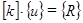,                                                            (1)

where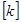is the stiffness matrix of the FEM,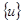is the nodal displacement vector, and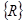is the vector of the nodal forces.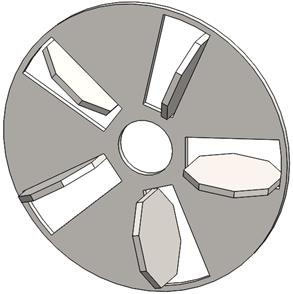Fig. 1. Geometric model of a pneumatic flexible shaft coupling

Having solved the system in (1), we find the displacement, after which we find stressand strain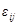using the strain-displacement relations (Cauchy) and stress-strain relations (Hooke’s law).

The application of the FEM involves the simulation of the geometry of a structure and its meshing into finite elements, the formation of a global stiffness matrix and solving large systems of linear equations (5,000÷500,000). The above problems have prompted the authors to apply the Salome-Meca platform-based, open-source Code_Aster application as a tool of numerical simulation. Code_Aster software allowed for the analysis of the SSS of the pneumatic flexible shaft coupling, taking into account the features and modes of operation.

3. RESULTS OF NUMERICAL EXPERIMENTS

The study focused on a numerical calculation of the SSS of a pneumatic flexible shaft coupling with the overall dimensions of 2,200 x 710 mm.

Prior to the simulation of a FEM, a solid-state spatial model of the pneumatic flexible shaft coupling (Fig. 1) was created using the KOMPAS-3D system for three-dimensional modelling. Taking into account the fact that the pneumatic flexible shaft coupling consists of two identical parts, the stress-strain analysis was performed on one part of the model.

The created solid-state model was then transferred to the Salome-Meca platform-based, open-source Code_Aster, which was used to set the boundary conditions, loads and areas where the load was applied, and to create a mesh of the FEM.

The boundary conditions were set so as to exclude the displacements of the pneumatic flexible shaft coupling as an absolutely rigid body. According to the working conditions, with the dynamic factor taken into account, the load on the pneumatic flexible shaft coupling was assumed to be 250 kN for every air spring. Fig. 2 presents the calculation schematic with the loads and boundary conditions indicated.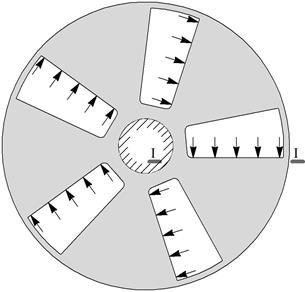a                                                                       b

Fig. 2. Calculation schematic (a) and FEM (b) of the pneumatic flexible shaft coupling

Three-dimensional finite elements with a maximum size of 0.120 m were used for the discretization of the pneumatic flexible shaft coupling as a spatial body. As a result of the discretization, a mesh of 14,489 tetrahedra and 4,534 nodes was obtained. The FEM of the pneumatic flexible shaft coupling is shown in Fig. 2b.

The material selected was DIN 1.1181 (C35E) steel with Young’s modulus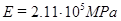, Poisson’s ratio ν=0.28, yield strength, and tensile strength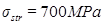.

As a result of a static calculation, the following SSS parameters of the pneumatic flexible shaft coupling were obtained: stress and strain along the corresponding axes; principal stresses and corresponding strains; equivalent stresses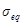calculated by Huber-Mises energy theory of strength; total displacements; displacements along corresponding axes; and factors of safety.

The results of the calculation show that the SSS of the pneumatic flexible shaft couplings is characterized by an uneven general and local distribution of stresses and displacements (Fig. 3). It has been established that high equivalent stresses (175 to 225 MPa) are observable in the areas located between the surface of the openings and the mounting surface for the shaft. This indicates that these areas need to be strengthened, that is, pneumatic flexible shaft couplings need to have a hub. Over a large area of the disc, the magnitudes of equivalent stresses are small and do not exceed 50 MPa. This indicates that the depth of the disc can be reduced. The maximum equivalent stresses calculated by the Huber-Mises theory are observed in the areas of abrupt changes in geometry (Fig. 3a). The maximum equivalent stresses occurring in the fillets reach 598 MPa, i.e.,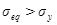. It should be noted that the maximum equivalent stresses occur within a small (in size) area (Fig. 3b), that is, they are localized and do not change throughout the depth of the disc.a                                                                       b

Fig. 3. Iso-surfaces of equivalent stresses, MPa (a) and the area of maximum equivalent stresses (b)

The study has also revealed the following:

- A dependence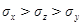of normal stresses was observed on a significant surface of the disc

- The values of tangential stresses are much lower than the values of normal stresses

- The values of tangential stresses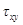are higher than the values of tangential stresses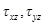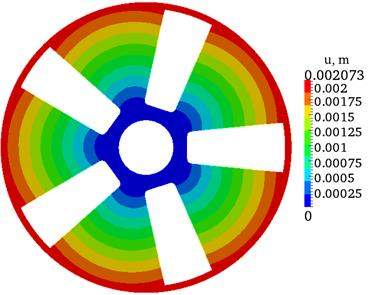Fig. 4. Iso-surfaces of total displacements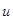The total displacement u diagram, shown in Fig. 4, indicates that total displacements vary linearly and equally over the entire surface, with the maximum total displacement (2 mm) on the rim of the disc, while the minimum total displacement is observed on the shaft mounting surface. The perforated trapezoidal openings slightly distort a circular distribution of total displacements.

The study shows that the maximum normal stresses (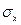,,), observed in zones with regular geometry and in zones of sharp geometry change, do not exceed the permissible stress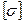for the selected material. These findings allow for changing the geometry of the disc of the pneumatic flexible shaft coupling so as to reduce its weight. The authors have proposed a pneumatic flexible shaft coupling, in which the thickness of the disc was reduced to 20 mm in the areas between the holes (Fig. 5). To assess the strength of the proposed disc of the pneumatic flexible shaft coupling, an analysis of the stress fields, strains and safety factors n was performed. It was found that the stresses hardly changed.

The analysis of the n diagram of the proposed disc of the pneumatic flexible shaft coupling (Fig. 6) shows that the values of factors of safety ranged from 1.15 to 11.1.

Thus, the study indicates that the strength and stiffness of the proposed disc of the pneumatic flexible shaft coupling are ensured. The changes in geometry allowed for reducing the mass of the disc by almost 30%.

An analysis of the experimental data (Fig. 3) revealed that the maximum normal stresses are observed in areas of sharp change in geometry (in areas of stress concentrators) and in the lower fibres of the curved surface of the disc, which are located next to the trapezoid openings. Reducing the maximum stresses in zones of sharp change in geometry is achieved by smooth transitions from one surface to another.Fig. 5. Safety factor diagram of the proposed disc of the pneumatic flexible shaft coupling

To determine the maximum stresses, we have allocated a part of the disc, which was located between two trapezoid openings. The selected part of the disc can be considered as a large curvature beam (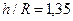), which undergoes pure bending (Fig. 6).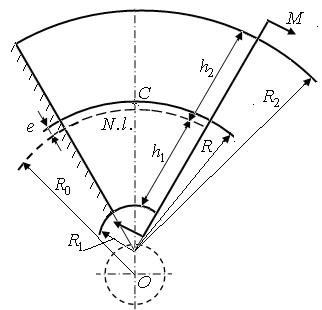`Fig. 6. Calculation scheme of the beam of great curvature`

`To determine the normal stresses in the selected part, the positions of the neutral layer were first determined by the following formula :`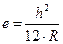,                                                               (2)

`where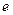is the distance between the neutral layer and the layer passing through the centres of gravity of the sections of the beam,is the height of the curvilinear beam, and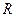is the radius of the layer passing through the centre of gravity of the sections of the curvilinear part.`
`The radiuswas determined by the formula below:`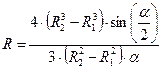,                                                  (3)

`where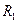andare the radii of curvature of the inner and outer fibres, respectively, and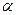is the angle formed by the creations carried through the major sides of the trapezoid openings.`
`Substituting the values of the radii and the angle (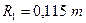,,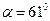) in (3), we find that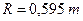.`
`The resulting valueand heightof the curvilinear beam (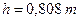) are substituted in (2) and we find that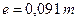. It should be noted that the position of the neutral layer in the global coordinate system, whose centre is located in the centre of the shaft on which the disc is planted, is determined by the radius, the value of which is equal to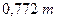. From Fig. 7, constructed based on the results of finite element analysis, it is clear that. Thus, the difference between the values obtained by two methods does not exceed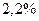.`
`To calculate the strength, it is necessary to always determine the greatest stresses that arise in the cross section. In the curvilinear beam, the largest absolute magnitudes of normal stress occur in the extreme fibres of the cross section, which are located on the curved surface of the beam. We wish to draw attention to the fact that the largest absolute magnitudes of normal stress in the muff disc also arise in the extreme fibres located on the curved surface of the beam (Fig. 3а). To determine such stresses, we apply a known formula, i.e.:`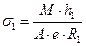,                                                            (4)

whereis the bending moment in the cross section,is the distance between the neutral line and the inner fibre (Fig. 6),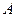is the cross-sectional area, andis the radius of curvature of the internal fibre of the curved beam.

`Substituting the values of all the quantities in (4) (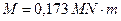,,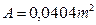,), we find that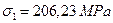. From Fig. 7, constructed based on the results of finite element analysis, it is clear that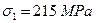. Thus, the difference between the values obtained by the two methods does not exceed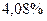.`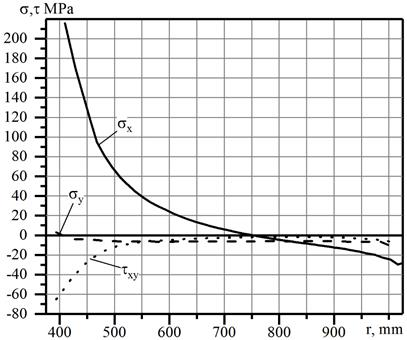`Fig. 7. Graph showing the variation in the normal and tangential stresses in Section I-I`

Consequently, the calculations revealed that the values of the obtained values and by the formulas in (2) and (4) almost coincide with the values of those obtained by the finite element method. This indicates that the part of the coupling that is limited by two trapezoid openings can be considered as a beam of large curvature and, in the first stage of designing the formulas in (2) and (4), used to determine the maximum normal stresses on which to determine the required sizes. After the first stage of the design, it is necessary to conduct a thorough finite element analysis of the SSS and, based on this analysis, select the final shape and dimensions of the coupling.

4. CONCLUSIONS

The SSS of pneumatic flexible shaft couplings, which was analysed by means of finite element modelling, is characterized by an uneven general and local distribution of stress. Changes in the total displacement were of a similar nature over the entire surface of the pneumatic flexible shaft coupling. The results of the SSS analysis enabled us to identify areas of maximum stresses in pneumatic flexible shaft couplings, which proved to be the areas of abrupt changes in the geometry. It has been found that the values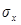andare higher than the values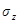. It has been established that the values of the tangential stressesare higher than the values,. The study allowed for changing the geometry and reducing the mass of the disc of the pneumatic flexible shaft coupling with a slight change in stresses and strains.
The study also confirmed that, in determining the stresses in the parts of the couplings located between the two holes, it is possible to use the formulas for the large curvature bars, with which these parts can be replaced. The findings will have a practical application in designing new pneumatic flexible shaft couplings.

References

2.             Homišin J. 2016. Characteristics of pneumatic tuners of torsional oscillation as a result of patent activity”. Acta Mechanica et Automatica 10(4): 316-323. ISSN: 1898-4088. DOI: 10.1515/ama-2016-0050.

3.             Годжаев З.А., A.A. Поповский, C.B. Гончаренко 2011. “Исследование характеристик пневматического упругого элемента рукавного типа в зависимости от давления воздуха, хода и формы поршня”. Вісник СевНТУ 120: 306-311. ISSN: 2307-6488. [In Russian: Godjayev Z.А., A.A. Popovskiy, S.V. Goncharenko. 2011. “Research on the performance of the pneumatic elastic sleeve element depending on air pressure, piston stroke and form”. Visnik SevNTU 120: 306-311. ISSN: 2307-6488.]

4.             Homišin J., P. Kaššay, M. Puškár, R. Grega, J. Krajňák, M. Urbanský, M. Moravič. 2016. Continuous tuning of ship propulsion system by means of pneumatic tuner of torsional oscillation”. International Journal of Maritime Engineering: Transactions of The Royal Institution of Naval Architects 158(A3): 231-238. ISSN: 1479-8751. DOI: 10.3940/rina.ijme.2016.a3.378.

5.             Поляков В.С., И. Д. Барбаш, О.А Ряховский. 1974. Справочник по муфтам. Ленинград, СССР: «Машиностроение». [In Russian: Polyakov V.S., I.D. Barbash, O.A. Ryakhovsky. 1974. Handbook of Couplings. Leningrad, USSR: “Mechanical Engineering”.]

6.             Виноградов, Б.В. 2012. “Проблемы создания двухдвигательных приводов барабанных мельниц”. Науковий вісник Національного гірничого університету 5: 61-65. ISSN: 2071-2227. [In Ukrainian: Vinogradov, B.V. 2012. “Problems in the creation of a two-motor drive tumbling mill”. Scientific Bulletin of National Mining University 5: 61-65. ISSN: 2071-2227.]

7.             Lušić Z., S. Kos, S. Krile. 2008. „Structural Analysis of Positioning Methods at Sea”. Nase More 55(1-2): 3-17.

8.             Gąska D., T. Haniszewski, 2017. „Numerical Modelling of I-Beam Jib Crane with Local Stresses in Wheel Supporting Flanges - Influence of Hoisting Speed”. Nase More 64(1): 7-13.

9.             Gąska D., T. Haniszewski, J. Margielewicz. 2017. "I-beam girders dimensioning with numerical modelling of local stresses in wheel-supporting flanges". Mechanika 23(3): 347-352. ISSN 1392-1207.

10.         Weskamp C., A. Koberstein, F. Schwartz, L. Suhl, S. Voss. 2018. “A two-stage stochastic programming approach for identifying optimal postponement strategies in supply chains with uncertain demand”. Omega. DOI: 10.1016/j.omega.2018.02.008. ISSN: 0305-0483.

11.         Wittek A.M., D. Gąska, B. Łazarz, T. Matyja. 2014. "Automotive stabilizer bar - stabilizer bar strength calculations using FEM, ovalization of radial areas of tubular stabilizer bars". Mechanika 20(6): 535-542. ISSN 1392-1207.

Received 04.03.2018; accepted in revised form 02.06.2018